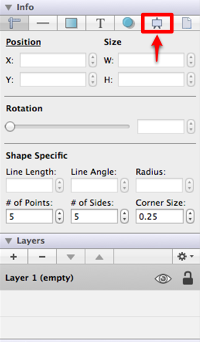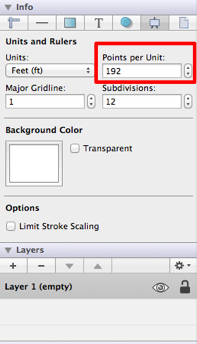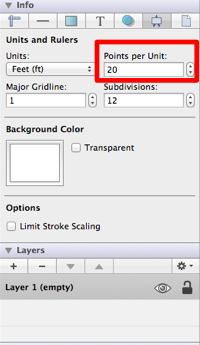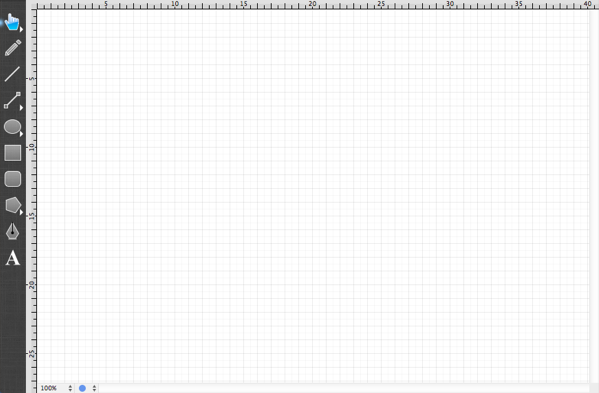# Step 3 - Configure Points Per Unit

After the Unit of Measure has been changed, the drawing canvas will have 12 subdivisions for each inch in feet rather than the original 8 subdivisions shown for inches. This is appropriate; however, at 100% zoom only (approximately) 5’ x 3’ are visible on the screen.The scale of the drawing must be adjusted before beginning to draw the floor plan. In TouchDraw for Mac this is (currently) done through the Points Per Unit option, which is accessed by clicking on the Canvas Tab in the Info Old section of the Sidebar Old as performed in the previous step.The Points per Unit option is highlighted in the above screenshot. Simple math is necessary to calculate the appropriate value for this setting.

First, the screen resolution used in this Mac example is 1024 x 768.

Second, the floor plan being drawn is 30’ x 30’.

Third, taking into consideration that a little bit of a margin will be wanted on the side of the drawing, the drawing view should be approximately 50 feet wide.

Fourth, the appropriate Points per Unit can be calculated by dividing 1024 (the number of horizontal pixels in the screen) by 50 (the width of the drawing in feet).

This calculation supplies a value of 20.48; however, we can simply round to the nearest integer and use 20 for setting the Points Per Unit. If a greater screen resolution will be used such as 1449 x 900, simply divide the first number by 50 to obtain the correct Points Per Unit value, which provides the value of 28.98 or 29 (rounded up). For the sake of simplicity, the traditional screen resolution of 1024 x 768 is used in this tutorial.The drawing canvas will appear as below once the Points Per Unit option has been changed.A future version of TouchDraw will perform the above calculation automatically by allowing the scale to be selected from a list.

Continue to Next Step